Next: Celestial Coordinate Systems
Up: Vectors and Matrices
Previous: Vectors and Matrices

### Using vectors

SLALIB provides conversions between spherical and vector form (sla_CS2C, sla_CC2S etc.), plus an assortment of standard vector and matrix operations (sla_VDV, sla_MXV etc.). There are also routines (sla_EULER etc.) for creating a rotation matrix from three Euler angles (successive rotations about specified Cartesian axes). Instead of Euler angles, a rotation matrix can be expressed as an axial vector (the pole of the rotation, and the amount of rotation), and routines are provided for this (sla_AV2M, sla_M2AV etc.).

Here is an example where spherical coordinates P1 and Q1 undergo a coordinate transformation and become P2 and Q2; the transformation consists of a rotation of the coordinate system through angles A, B and C about the z, new y and new z axes respectively:

            REAL A,B,C,R(3,3),P1,Q1,V1(3),V2(3),P2,Q2
:
*  Create rotation matrix
CALL sla_EULER('ZYZ',A,B,C,R)

*  Transform position (P,Q) from spherical to Cartesian
CALL sla_CS2C(P1,Q1,V1)

*  Multiply by rotation matrix
CALL sla_MXV(R,V1,V2)

*  Back to spherical
CALL sla_CC2S(V2,P2,Q2)


Small adjustments to the direction of a position vector are often most conveniently described in terms of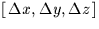. Adding the correction vector needs careful handling if the position vector is to remain of length unity, an advisable precaution which ensures that the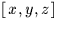components are always available to mean the cosines of the angles between the vector and the axis concerned. Two types of shifts are commonly used, the first where a small vector of arbitrary direction is added to the unit vector, and the second where there is a displacement in the latitude coordinate (declination, elevation etc.) alone.

For a shift produced by adding a smallvector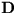to a unit vector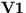, the resulting vector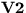has direction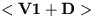but is no longer of unit length. A better approximation is available if the result is multiplied by a scaling factor of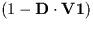, where the dot means scalar product. In Fortran:

            F = (1D0-(DX*V1X+DY*V1Y+DZ*V1Z))
V2X = F*(V1X+DX)
V2Y = F*(V1Y+DY)
V2Z = F*(V1Z+DZ)


The correction for diurnal aberration (discussed later) is an example of this form of shift.

As an example of the second kind of displacement we will apply a small change in elevation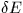to an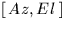direction vector. The direction of the result can be obtained by making the allowable approximation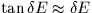and adding a adjustment vector of lengthnormal to the direction vector in the vertical plane containing the direction vector. The z-component of the adjustment vector is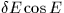,and the horizontal component is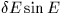which has then to be resolved into x and y in proportion to their current sizes. To approximate a unit vector more closely, a correction factor of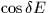can then be applied, which is nearly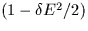for small. Expressed in Fortran, for initial vector V1X,V1Y,V1Z, change in elevation DEL (+ve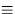upwards), and result vector V2X,V2Y,V2Z:

            COSDEL = 1D0-DEL*DEL/2D0
R1 = SQRT(V1X*V1X+V1Y*V1Y)
F = COSDEL*(R1-DEL*V1Z)/R1
V2X = F*V1X
V2Y = F*V1Y
V2Z = COSDEL*(V1Z+DEL*R1)


An example of this type of shift is the correction for atmospheric refraction (see later). Depending on the relationship betweenand E, special handling at the pole (the zenith for our example) may be required.

SLALIB includes routines for the case where both a position and a velocity are involved. The routines sla_CS2C6 and sla_CC62S convert from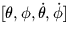to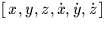and back; sla_DCS26 and sla_DC62S are double precision equivalents.Next: Celestial Coordinate Systems
Up: Vectors and Matrices
Previous: Vectors and Matrices

SLALIB --- Positional Astronomy Library